Next: Implementation Up: Newton method Previous: Newton method   Contents

Mathematical background

Let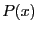be an univariate polynomial of degree: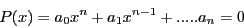with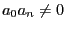,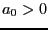. Newton theorem state that if it exists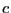such that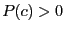and for all the derivative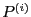of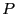, with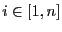, thenis an upper bound of the positive roots of. To findthe following scheme can be used:
1. letbe such that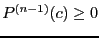2. let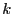the smallest integer such that either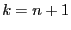or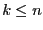and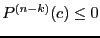3. ifthen substituteby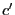such that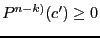and go to 2
4. returnA consequence of Newton theorem is that the best bound cannot be lower than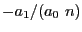.

Jean-Pierre Merlet 2012-12-20